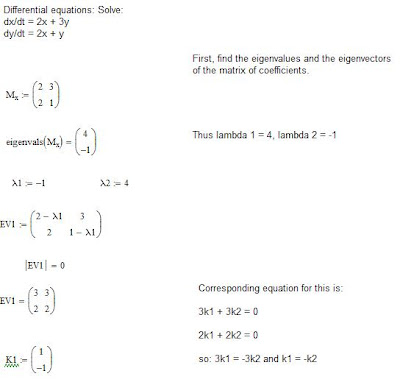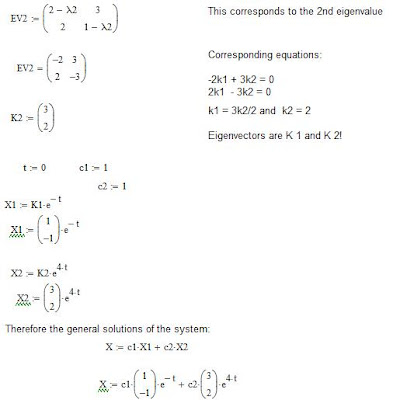## Wednesday, January 5, 2011

### Differential Equations: Homogeneous Linear SystemsIt's been a while since we did math, so let's return to the always interesting field of differential equations, and specifically: homogeneous linear systems. As before, a "linear" system refers to the degree and in this case, of one.

Now, to define homogeneous, let us consider the system as shown below:

dx1/dt = a11(t)x1 + a12(t)x2 + ..a1n(t)xn + f1(t)

dx2/dt = a21(t)x1 + a22(t)x2 + ..a2n(t)xn + f2(t)
:
:
:
dxn/dt = an1(t)x1 + an2(t)x2 + ..ann(t) xn + fn(t)

where the coefficients aij and the fi are functions continuous on a common interval, I.

Now, when fi(t) = 0, and i = 0, 1, 2.......n, this system is said to be homogeneous. Otherwise, it is called non-homogeneous.

Now, key to solving such a system is that one can use matrix form to do so (recall past blogs on matrices from last year) and in partricular something called "the fundamental matrix".

If then, X1, X2....X3 is a fundamental set of solutions of the homogenous system:

X' = AX

on an interval, I, then its general solution on the interval is:

X = c1X1 + c2 X2 + .......+ cn Xn

= c1[xi1j] + c2 [xi2j] + ...... cn [xinj]

where the brackets enfold row vectors

Now, let:

X1 = [xi1j] and X2 =[xi2j] and Xn = [xinj]

be a fundamental set of n solution vectors of the homogeneous system X' = AX on an interval I.

Then the matrix:

M(t) =

(x11.....x12........x1n)

(x21.....x22........x2n)

(xn1.....xn2........xnn)

is said to be a fundamental matrix of the system on the interval.

Now, let's proceed to use an example, by solving the homogeneous system:

dx/dt = 2x + 3y

dy/dt = 2x + y

Then the solution is straightforward (using matrices) and is shown in the graphic boxes (from the top to the one below).

Note the steps:

1) Find the eigenvalues from the matrix composed of coefficients

2) Having obtained the eigenvalues, obtain the first set of eigenvectors (EV1) as shown

3) Obtain the corresponding equation (in k1, k2) for the first set of eigenvectors

4) Thereby obtain the second eigenvalues and eigenvectors and the corresponding equations.

5) Note the eigenvectors are K1 and K2 at the end.

6) Write the general solutions (X1, X2) in terms of K1, K2.

7) Thence, write the general solutions of the system, viz.

X = c1 X1 + c2 X2

and note how the exponential (exp) form is obtained, as well as the vector rows for X1, X2.